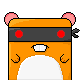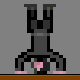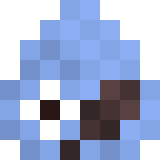Allegro.cc Forums » The Depot » 2007 Allegro ScreenHack, 80x25 compo

 This thread is locked; no one can reply to it.2007 Allegro ScreenHack, 80x25 compo
 kenmasters1976 Member #8,794 July 2007 Well, it was hard enough for me to make this 80x25 code. Anything under that is obviously out of my league.
 Matthew Leverton Supreme Loser January 1999The 20 line competition was different because it went by semicolons. Some people cheated by using commas as semicolons, but I avoided that.http://www.matthewleverton.com/misc/20lines.htmlI think 80x25 is a harder challenge simply because there is a finite limit to the number of characters you can use. (Compare that to my line 7, which is quite long.)
Næssén
Member #5,025
September 2004And here is my and Ted's entry for the competition. For you who haven't played Cursor*10 the game probably needs some instructions. The goal of the game is to get as high score as possible.

• Blue boxes give score points when clicked and unlocks the door to the next level

• Red boxes are closed doors

• Green boxes are open doors

• Yellow boxes are extra lives

• Red circles open doors when pushed

• Black boxes are openings to the previous level, however you can't use them to go back.

At most levels you need to click more than one blue box and/or push more than one red circle in order to unlock the door to the next level. It may seem tough at first, but you'll get help as the game progresses.

Oh, and one more thing, when the time is up you loose a life and have to restart from the first level.

 1 #include /////////////////Cursor*n v1.2 By Olof Naessén and Ted Steen 2 #define F(i,j)for(i=0;i=1;F(i,j)F(k,p 8 )F(r,p)D[r][k]=-1;F(i,j){r=rand()%p;k=(rand()%9)+1;Q(i,r,k,0,0);*D[i+1][r] 9 [k]=3;F(a,rand()%(i+1)+i/2)Q(i,rand()%p,(rand()%9)+1,1,rand()%((i/2)+2)+1);F(a, 10 rand()%2)if(i>5)Q(i,rand()%p,(rand()%9)+1,4,0);}}void v(int c){F(i,j){o=1;F(k,p) 11 {F(r,p){u=D[r][k];if(*u==0){a=r;b=k;if(i==c)if(u==0)R(r,k,4)else R(r,k,p)} 12 if(*u==1)if(u!=0){o=0;if(i==c){R(r,k,3)P(r*f+18,k*f+18,"%d",u)}}if(*u==3&c 13 ==i)R(r,k,0)if(*u==4){if(u==0){o=0;w=4;}else w=p;if(i==c)circlefill(B,r*f+20, 14 k*f+20,20,w);}if(i==c&&E[r][k]==1)R(r,k,14)}}D[a]=o;}}void main(){S( 15 );F(i,j)E[rand()%p][rand()%9+1]=1;allegro_init();text_mode(-1);install_mouse( 16 );install_keyboard();set_gfx_mode(2,s,s,0,0);B=create_bitmap(s,s);A[g-1][d]=0 17 ;while(!key){if(d>q){l--;g++;d=0;S();if(l==0){P(155,200,"Game Over",0)P(100, 18 209,"Highest level reached %d",h)}else rest(q);}if(l!=0){clear_to_color(B,15);P( 19 9,19,"Score %d",t)P(9,29,"Lives %d",l)P(150,9,"Time %d",q-d)e=A[g-1][d];v(C[g-1] 20 );*e=mouse_x;e=mouse_y;e=0;if(mouse_b)e=1;if(!mouse_b&&A[g-1][d-1]){ 21 e=0;e=1;}F(i,j)F(r,p)F(k,p){u=D[r][k];if(*u==4)u=0;}F(i,g){u=A[d] 22 ;r=*u/f;k=u/f;o=C;if(C==C[g-1])draw_sprite(B,mouse_sprite,*u,u);if(i 23 ==g-1)circlefill(B,*u,u,4,4);e=D[o][r][k];if(*e==4&&u)e=1;if(u){if(* 24 e==0&&e==1){C++;h=MAX(h,C);}if(*e==1&&e!=0){e--;t++;}u=&E[o][r][k 25 ];if(*u==1){*u=0;l++;}}}d++;}blit(B,screen,0,0,0,0,s,s);rest(9);}}END_OF_MAIN();

Edit: We managed to squeeze in a small pause between rounds.
Edit: Yet another update. We now have a Game Over message where you can see the highest level reached.
Edit: Fixed a MinGW issue, the code should now compile with MinGW.

_____________________

 MiquelFire Member #3,110 January 2003@kenmasters1976: Dude, that has maybe the most awesome bug/misfeature I have ever seen in a Tetris game! Don't like the piece you got, just toss it off to a side. ---Febreze (and other air fresheners actually) is just below perfumes/colognes, and that's just below dead skunks in terms of smells that offend my nose.MiquelFire.red | +MeIf it doesn't resolve the issue, we'll evaluate whether the latest round of anti-speculative-execution patches we've had to apply could somehow be responsible. (The performance impact of this fix is estimated to be just a couple of percent, but a banana on a football field isn't even that much and yet woe unto the quarterback who steps on it.) Gonna have to start looking at AMD CPUs. ~Owner of Nearly Free Speech.net
 Vanneto Member #8,643 May 2007 I am currently enjoying some sun in Croatia.I already made something before I left, I'll post it when I come back in Saturday.One question, must all of the 80x25 space be filled with code or can there be gaps in between? I'm just wondering. In capitalist America bank robs you.
 Kikaru Member #7,616 August 2006Use comments like this: ```codecodeblah//comment! ``` or ```codecodeblah///////// ``` to pad your lines. Ideally, the finished program's code will all be in a rectangle, 80 characters wide by 25 lines high. Or, if you wish, less than 25 lines high.
 kenmasters1976 Member #8,794 July 2007 Quote: @kenmasters1976: Dude, that has maybe the most awesome bug/misfeature I have ever seen in a Tetris game! Don't like the piece you got, just toss it off to a side. I know... I ran out of space for coding.I may try to fix that later... maybe.
 Neil Black Member #7,867 October 2006I've only managed to compile the tetris game, the rest give me errors.
Seppl
Member #4,656
May 2004Now with deallocation of the buffer bitmap.
This should compile. As a cpp file btw.
Hmm... 5 lines left. Maybe I'll add a highscore list.

 1 #include /////cpp file///////////////////////////by Seppl/////////// 2 BITMAP*B;int w,tt,i,ts=0;volatile int t=0;void i_t(){t++;}END_OF_FUNCTION(i_t() 3 )class C{public:double x,y,vx,vy;void L(){x+=vx;y+=vy;vy*=.95;}};class P:public 4 C{public:double r;void L(){C::L();vx*=.95;}P(){r=4;x=200;y=150;vx=vy=0;}void D( 5 ){circlefill(B,x,y,r,w);}}p;class E:public C{public:void L(){C::L();vx*=.98f;if 6 (((vx>0&&((x>p.x+p.vx&&x+vxp.x+p.vx&&xp.x)||(x+vxp.x))))&&yp.y){p.x=x+vx;p.vx=-p.vx+vx 8 *2;}if(x>400){y=rand()%280;x=400;vx=-(rand()%21+85)*.1f;}if(x<0){y=rand()%280;x 9 =0;vx=(rand()%21+85)*.1f;}}E(){x=-1;vy=0;}void D(){rectfill(B,x,y,x+vx,y+20,w); 10 }};void main(){E e;allegro_init();install_keyboard();set_color_depth(32)// 11 ;set_gfx_mode(GFX_AUTODETECT_WINDOWED,400,300,0,0);install_timer();bool go=1;// 12 LOCK_FUNCTION(i_t);LOCK_VARIABLE(t);install_int(i_t,20);B=create_bitmap(400,300 13 );w=makecol(255,255,255);tt=0;while(!key[KEY_ESC]&&go){clear(B);p.D();for(i=0;i 14 400||p.x<0||p.y<0||p.y>300)go=0;}}clear(B);textprintf_ex(B// 19 ,font,20,50,w,-1,"game over score: %i",tt);blit(B,screen,0,0,0,0,400,300) 20 ;clear_keybuf();while(!key[KEY_ESC]);destroy_bitmap(B);}END_OF_MAIN()//////////

EDIT:
Every entry here compiles fine for me.

__________________________________
All you need is lunch - The Rutles

Næssén
Member #5,025
September 2004We didn't think we would be able to squeeze more stuff into our game, but we did! We added some eye candy in the form of a shake effect when clicking boxes.

Also, I've attached a pre-compiled binary. See my previous post for game instructions.

 1 #include /////////////////Cursor*n v1.3 By Olof Naessén and Ted Steen 2 #define F(i,j)for(i=0;i[r][k];if(*u==0){a=r;b=k;if(i==c)if(u 8 ==0)R(r,k,4);else R(r,k,p);}if(*u==1)if(u!=0){o=0;if(i==c){if(u>0){X=-u 9 +L(u*2);Y=-u+L(u*2);u--;}R(r,k,3);z="%d";P(r*f+18,k*f+18,u);X=Y=0 10 ;}}if(*u==4){if(u==0){o=0;w=4;}else w=p;if(i==c)circlefill(B,r*f+20,k*f+20,20 11 ,w);}if(*u==3&c==i)R(r,k,0);if(i==c&&E[r][k]==1)R(r,k,14);}}D[a]=o;} 12 }*S(){srand(3);F(i,j)F(k,p)F(r,p)*D[r][k]=-1;F(i,j){C=1;r=L(p);k=L(9)+1;Q( 13 i,r,k,0,0);*D[i+1][r][k]=3;F(a,L(i+1)+i/2)Q(i,L(p),L(9)+1,1,L((i/2)+2)+1);F(a,L( 14 2))if(i>5)Q(i,L(p),L(9)+1,4,0);}}*main(){allegro_init();S();F(i,j)E[L(p)][L(9 15 )+1]=1;text_mode(-1);install_mouse();install_keyboard();set_gfx_mode(2,s,s,0,0); 16 B=create_bitmap(s,s);A[g-1][d]=0;while(!key){if(d>q){l--;g++;d=0;S();if(l 17 ==0){z="Game Over";P(155,200,0);z="Highest level reached %d";P(100,209,h);}else 18 rest(q);}if(l!=0){clear_to_color(B,15);z="Time %d";P(150,9,q-d);z="Score %d";P(9 19 ,19,t);z="Lives %d";P(9,29,l);e=A[g-1][d];v(C[g-1]);*e=mouse_x;e=mouse_y;e 20 =0;if(mouse_b)e=1;if(!mouse_b&&A[g-1][d-1]){e=0;e=1;}F(i,j)F(r,p)F(k 21 ,p){u=D[r][k];if(*u==4)u=0;}F(i,g){u=A[d];r=*u/f;k=u/f;o=C;if(C[i 22 ]==C[g-1])draw_sprite(B,mouse_sprite,*u,u);if(i==g-1)circlefill(B,*u,u,4,4 23 );e=D[o][r][k];if(*e==4&&u)e=1;if(u){if(*e==0&&e==1){C++;h=MAX(h, 24 C);}if(*e==1&&e!=0){e--;e+=4;t++;}u=&E[o][r][k];if(*u==1){*u=0;l++;} 25 }}d++;}blit(B,screen,0,0,0,0,s,s);rest(9);}}END_OF_MAIN()/////tinyurl.com/6l3ay7

Neil Black said:

I've only managed to compile the tetris game, the rest give me errors.

What was your error with our entry? The game has to be compiled with C, not C++.

_____________________

Arvidsson
Member #4,603
May 2004Wow, I actually finished something and it was fun too!

Move left and right with arrowkeys, use X to jump and double-jump in midair and Z to boost yourself even higher up in the air. You get the boosts when collecting the coins. You win when all coins are collected and you lose if you fall off the screen. Should compile in both C and C++. Oh and press R to generate a new level.

 1 #include ///////////////////////////////////BoxJump v2.0 by arvidsson 2 #define P(x,y,t,a) textprintf_ex(B,font,x,y,makecol(255,255,255),-1,t,a);/////// 3 #define Q(m,n) ((rand()%((m+1)-n)+n))/////////////////////////////////////////// 4 int w=640,t,x,y,b,c,n,i,j,k,m,q,o,p,z;float g; int C(int x1,int y1, 5 int w1,int h1,int x2,int y2,int w2,int h2){if((x1>x2+w2)||(y1>y2+h2)||(x2>x1+w1) 6 ||(y2>y1+h1))return 0;return 1;};void G(){n=j=g=z=0;x=Q(580,20);y=Q(40,0);for(i= 7 0;i<20;i++){V:b=Q(580,20);b=Q(580,20);k=0;do{if(k!=i)if(C(b,b[ 8 i],19,19,b[k],b[k],19,19))goto V;k++;}while(k=Q(580,20);c=1;for(k=0;k<20;k++){if(C(b[k],b[k][ 10 1],19,19,c-10,c-10,10,10))goto J;}}}void T(){t++;}void main(){srand( 11 time(0));allegro_init();install_keyboard();set_gfx_mode(2,w,w,0,0);install_int(T 12 ,20); BITMAP* B=create_bitmap(w,w); set_keyboard_rate(0,0);G(); while(!key){ 13 while(t>0){t--;if(keypressed()){i=readkey();if((i&0xff)=='x'&&j){g=-2;j--;} else 14 if((i&0xff)=='z'&&z){g=-5;z--;}}if(key)x-=2;if(key)x+=2;if(g>6)g=6;for(i 15 =0;i<20;i++){if(C(x,y,9,9,b,b,19,19)){m=MAX(x,b);q=MAX(y,b[ 16 1]);o=MIN(x+9,b+19);p=MIN(y+9,b+19);if(o-m>p-q){if(q==b){y=b[i 17 ]-10;j=2;g=0;}else{y=b+20;}}else{if(m==b){x=b-10;}else{x=b[ 18 i]+20;}}}}for(i=0;i<5;i++)if(C(x,y,9,9,c-5,c-5,9,9)&&c){c[i 19 ]=0;n++;z++;}if(x<0||x>640||y>640){P(300,300,"FAIL!",0);blit(B,screen,0,0,0,0 20 ,w,w);readkey();G();}if(n>=5){P(275,300,"YOU ARE WIN!",0);blit(B,screen,0,0,0,0, 21 w,w);readkey(); G();}g+=0.1;y+=g;if(key)G();}rect(B,x,y,x+9,y+9,makecol(255, 22 255,255));P(10,10,"Boost: %d",z);for(i=0;i<5;i++)if(c)circlefill(B,c 23 ,c,5,makecol(255,255,0)); for(i=0;i<20;i++)rect(B,b,b,b+ 24 19, b+19, makecol(0,0,255)); blit(B,screen,0,0,0,0,w,w); clear_bitmap(B);} 25 destroy_bitmap(B);}END_OF_MAIN()////////////////////////////////////////////////

{"name":"595181","src":"\/\/djungxnpq2nug.cloudfront.net\/image\/cache\/e\/7\/e703b0e31767c4718ab280baa62362f5.png","w":323,"h":336,"tn":"\/\/djungxnpq2nug.cloudfront.net\/image\/cache\/e\/7\/e703b0e31767c4718ab280baa62362f5"}edit: Oops, seems my entry is 79x25. Scite fooled me.
edit2: Updated my entry. It is now in 80x25 format and destroys the buffer like a good little hack. Didn't add anything new gameplay wise, just optimized the use of space a little. Added screenshot too!

 Jeff Bernard Member #6,698 December 2005Quote: It may seem tough at first, but you'll get help as the game progresses. Is the help those other cursors that appear? At first I thought I had lagged the game really bad or something because the second cursor appeared to do exactly what I had done on the first level. --I thought I was wrong once, but I was mistaken.
 BAF Member #2,981 December 2002I need an idea for a game to write.
 Ted Steen Member #9,736 April 2008Jeff Bernard said: Is the help those other cursors that appear? At first I thought I had lagged the game really bad or something because the second cursor appeared to do exactly what I had done on the first level. Yes, that's your mouse from the previous round helping you. You need to do some clever cooperation with yourself to get the high scores!The current world My record is 1510 points, I reached level 11. (see attachment)Edit:Now my best score is 2749. I didn't beat Jeff, but I beat Olofhttp://www.allegro.cc/files/attachment/595182
 Næssén Member #5,025 September 2004Ted Steen said: The current world record is 1510 points, I reached level 11. (see attachment) I beat your record Ted, 2720 points and I reached level 14! _____________________darkbits.org | Google+
 Jeff Bernard Member #6,698 December 2005Quote: I beat your record Ted, 2720 points and I reached level 14! pwned: 3139, level 15. http://www.allegro.cc/files/attachment/595179It may just be a quick hack, but it's a really fun game.;D --I thought I was wrong once, but I was mistaken.
kenmasters1976
Member #8,794
July 2007

Updated mine too. Tweaked the drawing code, reduced almost all variable names to single letter ones and replaced all Allegro constants with numbers to save space and have room to improve it.

I made the playfield 10 blocks wide as usual in Tetris games - this also fixed the problem that MiquelFire mentioned (and likely created new ones). Also added the features I couldn't include in the previous one and reduced window size to fit just the game area.

 1 #include // SHTetris.c 2 #define f() for(i=0;i<4;i++) ///////////// 3 #define B() blit(O,screen,0,0,0,0,640,480) 4 #define RF(c) rectfill(O,xo+j*16,yo+y*16,xo+j*16+16,yo+y*16+16,c) 5 int F;int ps={0,0,0,15,1,1,1,1,0,0,0,15,1,1,1,1,0,2,2,3,0,0,7,4,0,3, 6 1,1,0,0,1,7,0,1,1,3,0,0,4,7,0,3,2,2,0,0,7,1,0,1,3,2,0,0,6,3,0,1,3,2,0,0,6,3,0,2 7 ,3,1,0,0,3,6,0,2,3,1,0,0,3,6,0,0,7,2,0,1,3,1,0,0,2,7,0,2,3,2,0,0,3,3,0,0,3,3,0, 8 0,3,3,0,0,3,3};int i,j,p,q=0,m,x,y,w,a=0,o,no=0,d,e,g,k,lt,rt,ltl,rtl, cf,h, 9 cm,rp,cr;BITMAP *O;int UF(){for(i=23;i>=0;i--)if(F==0xFFFF){DL(0,0,i,0xFFFF, 10 0xFFFFFF,-1);B();rest(50);DL(0,0,i,0xFFFF,0x44A3FF,-1);B();rest(50);}for(i=23;i 11 >0;i--){if(F==0xFFFF)for(j=i;j>=1;j--)F[j]=F[j-1];}}int DL(int xo,int yo,int 12 y,int d,int c,int sc){for(j=0;j<16;j++)if(d&1<=0xE007;srand(time(NULL));while(!key){if(!a){p=q;q=rand()%7;o=no;no=rand 16 ()%4;d=0;a=1;x=6;y=3;w=6;e=10;g=32;}if(h>e){y++;h=0;}if(cm)x=w;rp=0;if(key) 17 {lt++;if(lt>ltl){lt=0;w=x-1;ltl/=2;if(ltl<4)ltl=4;}}if(key){rt++;if(rt>rtl) 18 {rt=0;w=x+1;rtl/=2;if(rtl<4)rtl=4;}}if(key&&!k){o=(o+1)%4;rp=1;k=1;}if(!key 19 )k=0;if(!key){ltl=32;lt=31;}if(!key){rtl=32;rt=31;}if(key){e=1; 20 g=1;}if(!key){e=10;g=32;}if(rp){f()m=ps[p][(o)*4+i];cr=1;f(){cr=cr&&((F[ 21 y-i]&(m)<=ps[p][(o)*4+i];cm=1; f()cm= 22 cm&&(F[y-1-i]&m<<g){f()F[y-i-1]|=m<, 24 0xFFA6A6,0xFFFFA9);f()DL(0,0,y-1-i,m<=ps[q][no*4 25 +i];DL(208,100,5-i,m,0x44A3FF,0xFFA6A6);}B();rest(15);}}END_OF_MAIN()

{"name":"595180","src":"\/\/djungxnpq2nug.cloudfront.net\/image\/cache\/5\/7\/57c86258f645a0ce3fed379913e2afa0.gif","w":261,"h":431,"tn":"\/\/djungxnpq2nug.cloudfront.net\/image\/cache\/5\/7\/57c86258f645a0ce3fed379913e2afa0"}Næssén Member #5,025 September 2004kenmasters1976, your tetris game is really starting to shape up. The only thing I miss is a score counter.Jeff Bernard said: 3139, level 15 I'm impressed. _____________________darkbits.org | Google+
 Ted Steen Member #9,736 April 2008Kenmasters1976, That is one squeezed game of tetris! but you could double the fun with a score counter!My suggestion is to replace these defines with functions instead. ```#define B() blit(O,screen,0,0,0,0,640,480) #define RF(c) rectfill(O,xo+j*16,yo+y*16,xo+j*16+16,yo+y*16+16,c) ``` will turn into something like this ```*B(){blit(O,screen,0,0,0,0,640,480);} *R(c){rectfill(O,xo+j*16,yo+y*16,xo+j*16+16,yo+y*16+16,c);} ``` Now you can almost fit them into one line.A few minor optimizations would be to replaceBITMAP *Owithint*OAnd replace ```int DL(int xo,int yo,int y,int d,int c,int sc) ``` with ```*DL(xo,yo,y,d,c,sc) ``` Now... that should be enough for a score counterArvidsson
Member #4,603
May 2004What the hell is wrong with me? Another entry, that is what's wrong with me.

ARE YOU UP TO THE TASK TO TAKE COMMAND AND DODGE FUNNY LOOKING ASTEROIDS?

Steer your spacecraft with arrowkeys. Reset with R. Quit with ESC. Every 15 seconds the difficulty level is increased which means more asteroids (or space balls if you'd like) to dodge. The maximum level is 10 which means 50 asteroidballs onscreen at ALL times. I almost made it to level 5, level 10 must be hell I suppose (only hardcore gamers need apply). The blue box on the ship (aka the cockpit) is the hit area.

 1 #include ////////////////////////////////SpaceDodge v1.0 by arvidsson 2 int w=400,i,j,x,y,c,l,q,z,t,u,k,s,e;R(m,n){return(rand()%(m+1-n))+ 3 n;}C(x1,y1,w1,h1,x2,y2,w2,h2){if((x1>x2+w2)||(y1>y2+h2)||(x2>x1+w1)||(y2>y1+h1)) 4 return 0;return 1;}void T(){t++;u++;}N(){l=1;c=u=q=k=z=0;for(i=0;i<75;i++){s[ 5 0]=R(400,0);s=R(400,0);s=R(5,2);j=s*51;s=makecol(j,j,j); 6 }for(i=0;i<50;i++)e=0;x=w/2;y=350;}main(){allegro_init();set_gfx_mode(2,w, 7 w,0,0); install_keyboard();install_int(T,20);BITMAP *B=create_bitmap(w,w);srand( 8 time(0));N();while(!key){while(t>0){t--;if(u>=50){k++;q++;u=0;}if(q>=15){l++ 9 ;q=0;}if(l>=10)l=10;for(i=0;i<75;i++){s+=s;if(s>w){s=0;s 10 =R(400,0);s=R(5,2);j=s*51;s=makecol(j,j,j);}}i=0;while(i 11 <50&&c){c++;e=1;e=makecol(R(255,0),R(255,0),R(255,0 12 ));int d=R(3,1);if(d==1){e=R(w,0);e=-10;e=R(2,0);e=R(5,1 13 );}if(d==2){e=-10;e=R(300,0);e=R(5,1);e=R(2,0);}if(d==3) 14 {e=410;e=R(300,0);e=-R(5,1);e=R(2,0);}}i++;}for(i=0;i<50 15 ;i++){if(e){e+=e;e+=e;}if(e<-20||e>420 16 ||e<-20||e>400){e=0;c--;}}for(i=0;i<50;i++){if(e)if(C(x- 17 2,y,4,5,e-5,e-5,5,5)){e=0;z=1;}}if(key)x-=4;if(key)x+= 18 4;if(key)y-=4;if(key)y+=4;if(key)N();if(x<10)x=10;if(x>390)x=390;if( 19 y<10)y=10;if(y>390)y=390;}while(!t)rest(1);for(i=0;i<75;i++)putpixel(B,s,s 20 ,s);for(i=0;i<50;i++)if(e)circlefill(B,e,e,5,e[ 21 4]);if(z)textprintf_centre_ex(B,font,w/2,w/2,15,-1,"GAME OVER!");if(!z){triangle 22 (B,x,y-10,x+10,y+10,x-10,y+10,15);rectfill(B,x-2,y,x+2,y+5,32);}textprintf_ex(B, 23 font,10,10,15,-1,"Level: %d Time: %d",l,k);blit(B,screen,0,0,0,0,w,w);//////woot 24 clear_bitmap(B);if(z){i=0;while(!i){clear_keybuf();j=readkey();if((j>>8)==59)/// 25 return;else if((j>>8)==18){N();i=1;}}}}destroy_bitmap(B);}END_OF_MAIN()/////////

{"name":"595186","src":"\/\/djungxnpq2nug.cloudfront.net\/image\/cache\/9\/0\/90c88f30bfdf23fdcc43fd926665e9aa.png","w":403,"h":430,"tn":"\/\/djungxnpq2nug.cloudfront.net\/image\/cache\/9\/0\/90c88f30bfdf23fdcc43fd926665e9aa"}This contest will be the end of me, I already have a new thingy I want to implement. Curses, programming is supposed to be boring, no?edit: I actually got to level 7 and discovered a cheat which is fixed now.

 Paul Pridham Member #250 April 2000Quote: *B(){blit(O,screen,0,0,0,0,640,480);}*R(c){rectfill(O,xo+j*16,yo+y*16,xo+j*16+16,yo+y*16+16,c);} Why is everyone prefixing their K&R-style default functions with a * ? I don't see the purpose... you're returning no value (especially not a pointer to int) and wasting a character in your code.
 Ted Steen Member #9,736 April 2008ppridham said: Why is everyone prefixing their K&R-style default functions with a * ? I don't see the purpose... you're returning no value (especially not a pointer to int) and wasting a character in your code. You are right, I just assumed that you needed to specify the return type, and * is smaller than void. C is not my "native language"Yay! Now we have four more characters to play with!! Olof - let's add network support!
 Kikaru Member #7,616 August 2006Urg, I had an idea, but it was really boring compared to these... I'm starting over now.Matthew Leverton
Supreme Loser
January 1999Tetris

{"name":"595191","src":"\/\/djungxnpq2nug.cloudfront.net\/image\/cache\/8\/9\/8975a23dbdf433e7ee0863aff039dacd.png","w":304,"h":388,"tn":"\/\/djungxnpq2nug.cloudfront.net\/image\/cache\/8\/9\/8975a23dbdf433e7ee0863aff039dacd"}Mokkan Member #4,355 February 2004Those of you making Tetris should figure out a way to use more color.I'd love to try this, but I'm not sure if I could come up with anything. Perhaps I'll give it a go anyway...